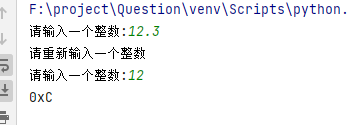2021-11-20 11:57

编写函数，将十进制数转化为十六进制数• 写回答
• 好问题 提建议
• 追加酬金
• 关注问题
• 收藏
• 邀请回答

1条回答默认 最新

•辉煌仪奇 2021-11-20 12:02
已采纳
def convert(n):
if not isinstance(n,int):
print("请重新输入一个整数")
return ""
_=[0,1,2,3,4,5,6,7,8,9,'A','B',"C","D","E","F"]
d = str(_[n % 16])
mark = n // 16
if mark > 0:
return convert(mark) + d
else:
return d
while True:
int10=eval(input("请输入一个整数:"))
hexnumber="0x"+convert(int10)
if len(hexnumber)!=2:
print(hexnumber)
break评论
解决 1 无用
打赏 举报 编辑记录